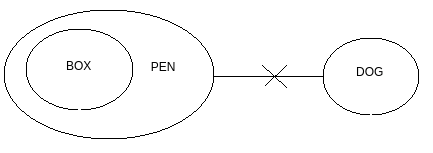Q

# Given two statements, verify the conclusions and mark the answer as given below.

Directions : Given two statements, verify the conclusions and mark the answer as given below.

Statements:

I.          All boxes are pens                    II.          No dogs are pens

Conclusions:

I.          No boxes are dogs                   II.          Some pen are boxes

• Option 1)

if only conclusion I follow.

• Option 2)

If only conclusion II follows.

• Option 3)

If either conclusion I or II follows.

• Option 4)

If neither of the two conclusions follows.

• Option 5)

If both conclusions follow

ViewsI. Follows

II. Follows

Exams
Articles
Questions